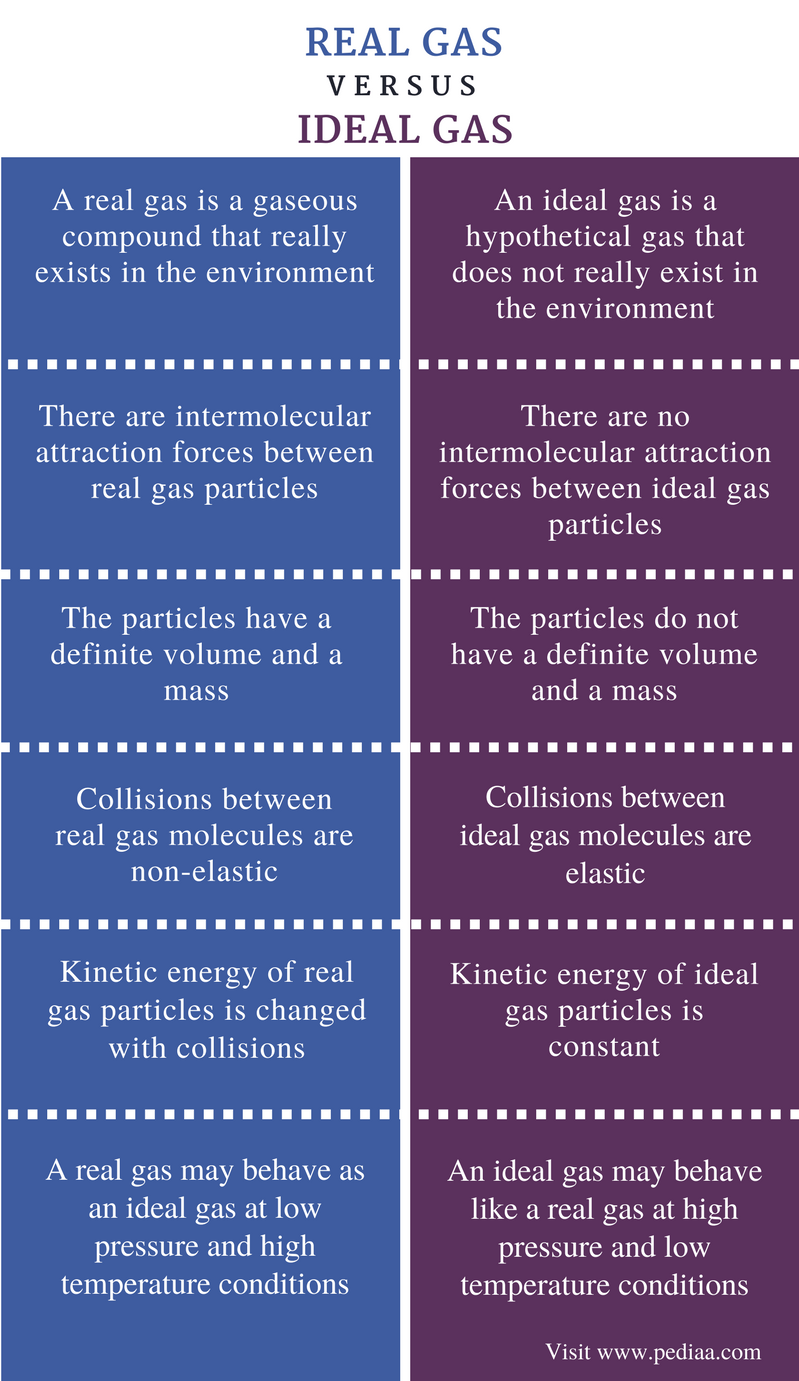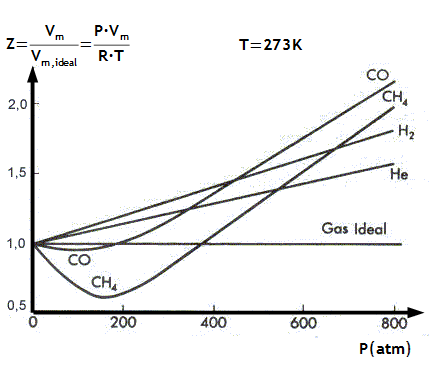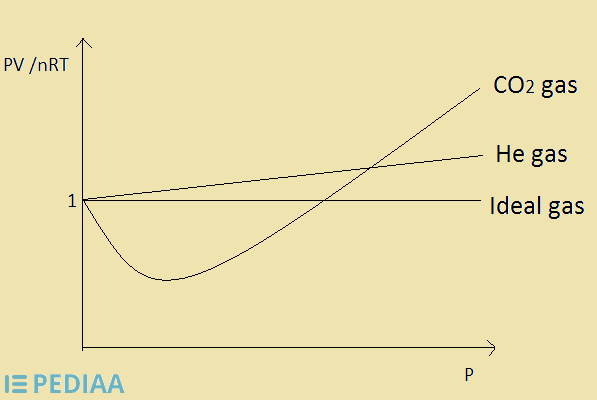# Difference Between Real and Ideal Gas

## Main Difference – Real vs Ideal Gas

A gas is a type of physical state that matter can exist in. When the particles or the molecules of a compound are free to move anywhere inside a container, this compound is called a gas. The gaseous state is different from other two physical states (solid and liquid state) according to the way particles or molecules are packed. A real gas is a gaseous compound that really exists. An ideal gas is a gaseous compound that does not exist in reality but is a hypothetical gas. However, some gaseous compounds show approximately similar behavior to that of ideal gases at a specific temperature and pressure conditions. Therefore, we can apply gas laws for that kind of real gases by assuming that they are ideal gases. Even though the proper conditions are provided, a real gas cannot become100% close to the behavior of an ideal gas due to the differences between real and ideal gas. The main difference between real and an ideal gas is that real gas molecules have intermolecular forces whereas an ideal gas has no intermolecular forces.

### Key Areas Covered

1. What is a Real Gas

– Definition, Specific Properties
2. What is an Ideal Gas

– Definition, Specific Properties
3. What is the Difference Between Real and Ideal Gas

– Comparison of Key Differences

Key Terms: Gas, Ideal Gas, Gas Laws, Intermolecular Forces, Real Gas## What is a Real Gas

A real gas is a gaseous compound that really exists in the environment. These real gases are composed of different atoms or molecules that are called particles. These gas particles are in constant motion. A gas particle has a definite volume and mass. Therefore, a gas has a definite volume and a mass. The volume of a gas is considered as the volume of the container in which the gas is kept in.

Some real gases are composed of atoms. For example, Helium gas is composed of Helium atoms. But other gases are composed of molecules. For example, Nitrogen gas is composed of N2 molecules. Therefore, these gases have a mass and a volume.

Furthermore, real gas molecules have intermolecular attractions between them. These attraction forces are called Van Der Waal interactions. These attraction forces are weak. Collisions between real gas molecules are non-elastic. This means when two real gas particles colloid with each other, a change in the energy of the particle and a change in the direction of its movement can be observed.

However, some real gases may behave as ideal gases under low pressure and high temperature conditions. At high temperatures, the kinetic energy of gas molecules is increased. Therefore the motion of gas molecules speed up. This results in less or no intermolecular interactions between real gas molecules.

Therefore, at low pressure and high temperature conditions, we can apply gas laws for real gases. For example, at low pressure and high temperature;

PV / nRT  ≈  1

Where  P is the pressure of the gas,

V is the volume of the gas,

n is the number of moles of gas,

R is the ideal gas constant and

T is the temperature of the system.

This value is called the compressibility factor. It is a value that is used as a correction factor for the deviation of a property of a real gas from an ideal gas. But for real gases PV ≠ nRT.Figure 1: Compressibility factor for different gases with respect to that of an ideal gas

Although the value of PV /nRT is not exactly equal to 1, it is an approximately equal value at low pressure and high temperature conditions.

## What is an Ideal Gas

An ideal gas is a hypothetical gas that does not really exist in the environment. The concept of ideal gas was introduced since the behavior of real gases are complicated and different from each other, and the behavior of a real gas can be described with respect to the properties of an ideal gas.

Ideal gases are gaseous compounds that are composed of very tiny molecules that have a negligible volume and a mass. As we already know, all real gases are composed of atoms or molecules that have a definite volume and a mass. The collisions between ideal gas molecules are elastic. This means, there are no changes in the kinetic energy or the direction of the movement of the gas particle.

There are no attraction forces between ideal gas particles. Therefore, particles move here and there freely. However, ideal gases may become real gases at high pressures and low temperatures since the gas particles come close to each other with a reduced kinetic energy that will result in the formation of intermolecular forces.Figure 2: The behavior of Ideal gas with respect to the He gas and CO2 gas

An ideal gas obeys all the gas laws without any assumptions. The value for PV /nRT for an ideal gas equals 1. Therefore the value for PV is equal to the value for nRT. If this value (compressibility factor) is equal to 1 for a particular gas, then it is an ideal gas.

## Difference Between Real and Ideal Gas

### Definition

Real Gas: A real gas is a gaseous compound that really exists in the environment.

Ideal Gas: An ideal gas is a hypothetical gas that does not really exist in the environment.

### Intermolecular Attractions

Real Gas: There are intermolecular attraction forces between real gas particles.

Ideal Gas: There are no intermolecular attraction forces between ideal gas particles.

### Gas Particle

Real Gas: The particles in a real gas have a definite volume and a mass.

Ideal Gas: The particles in an ideal gas do not have a definite volume and a mass.

### Collisions

Real Gas: Collisions between real gas molecules are non-elastic.

Ideal Gas: Collisions between ideal gas molecules are elastic.

### Kinetic Energy

Real Gas: The kinetic energy of real gas particles is changed with collisions.

Ideal Gas: The kinetic energy of ideal gas particles is constant.

### Change in the State

Real Gas: A real gas may behave as an ideal gas at low pressure and high temperature conditions.

Ideal Gas: An ideal gas may behave like a real gas at high pressure and low temperature conditions.

### Conclusion

Real gases are gaseous compounds that really exist in the environment. But ideal gases are hypothetical gases that do not really exist. These ideal gases can be used to understand the behavior of real gases. When applying a gas law for a real gas, we can assume that real gasses behave as ideal gasses at low pressure and high temperature conditions. But the accurate way is to use correction factors for the calculations rather than assuming. The correction factors are obtained by determining the difference between real and ideal gas.

##### References:

1. “Real Gases.” Chemistry LibreTexts, Libretexts, 1 Feb. 2016, Available here. Accessed 6 Sept. 2017.
2. “Compressibility factor.” Wikipedia, Wikimedia Foundation, 11 Aug. 2017, Available here. Accessed 6 Sept. 2017.
3. “Ideal gas.” Wikipedia, Wikimedia Foundation, 30 Aug. 2017, Available here. Accessed 6 Sept. 2017.

##### Image Courtesy:

1. “Factor Z vs” By Antoni Salvà – Own work (CC BY-SA 4.0) via Commons Wikimedia